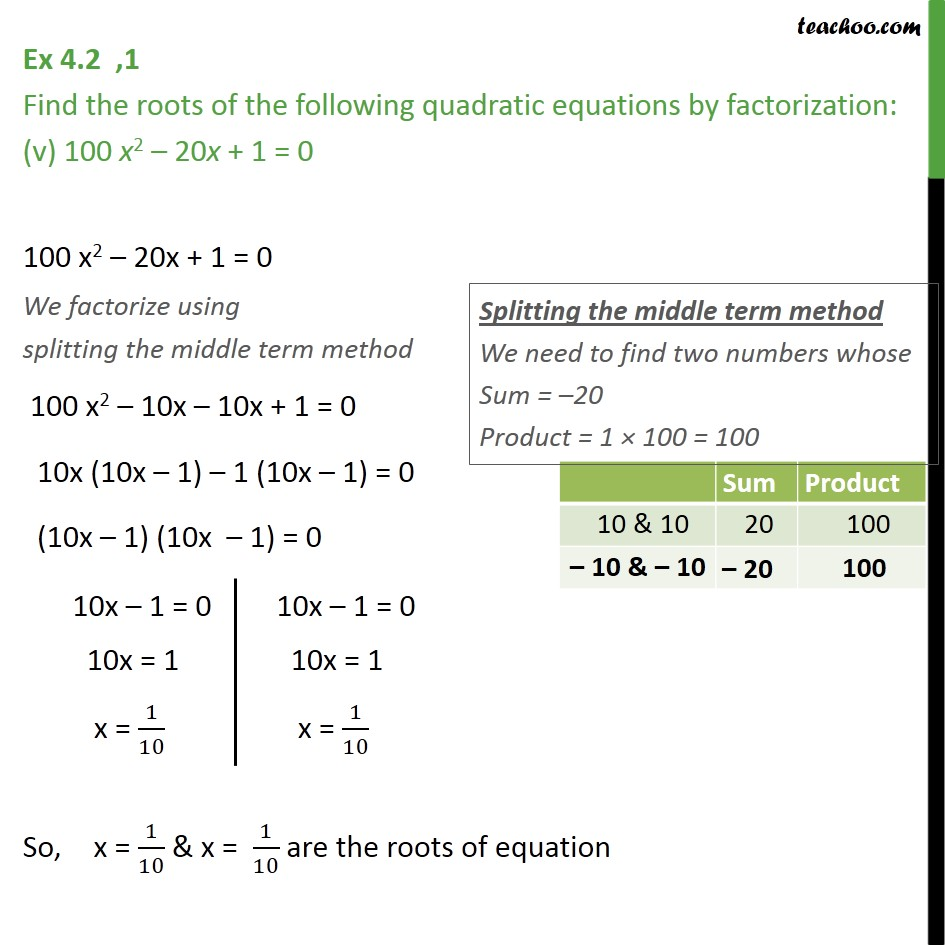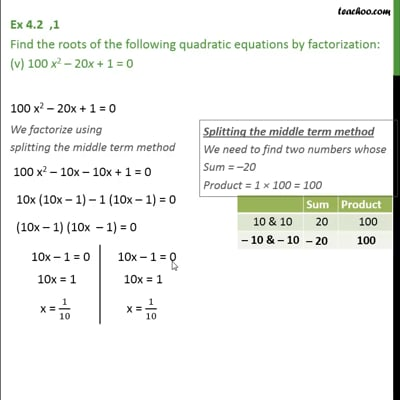Ex 4.2

Chapter 4 Class 10 Quadratic Equations
Serial order wiseThis video is only available for Teachoo black users

Introducing your new favourite teacher - Teachoo Black, at only ₹83 per month

### Transcript

Ex 4.2 ,1 Find the roots of the following quadratic equations by factorization: (v) 100 x2 20x + 1 = 0 100 x2 20x + 1 = 0 We factorize using splitting the middle term method 100 x2 10x 10x + 1 = 0 10x (10x 1) 1 (10x 1) = 0 (10x 1) (10x 1) = 0 So, x = 1/10 & x = 1/10 are the roots of equation